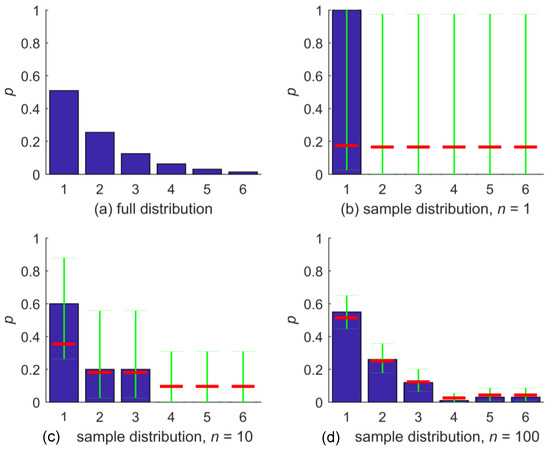Open AccessArticle

# A Maximum-Entropy Method to Estimate Discrete Distributions from Samples Ensuring Nonzero Probabilities

byPaul Darscheid 1,Anneli Guthke 2andUwe Ehret 1,*1
Institute of Water Resources and River Basin Management, Karlsruhe Institute of Technology—KIT, 76131 Karlsruhe, Germany
2
Institute for Modelling Hydraulic and Environmental Systems (IWS), University of Stuttgart, 70569 Stuttgart, Germany
*
Author to whom correspondence should be addressed.
Entropy 2018, 20(8), 601; https://doi.org/10.3390/e20080601
Received: 18 July 2018 / Revised: 9 August 2018 / Accepted: 13 August 2018 / Published: 13 August 2018
When constructing discrete (binned) distributions from samples of a data set, applications exist where it is desirable to assure that all bins of the sample distribution have nonzero probability. For example, if the sample distribution is part of a predictive model for which we require returning a response for the entire codomain, or if we use Kullback–Leibler divergence to measure the (dis-)agreement of the sample distribution and the original distribution of the variable, which, in the described case, is inconveniently infinite. Several sample-based distribution estimators exist which assure nonzero bin probability, such as adding one counter to each zero-probability bin of the sample histogram, adding a small probability to the sample pdf, smoothing methods such as Kernel-density smoothing, or Bayesian approaches based on the Dirichlet and Multinomial distribution. Here, we suggest and test an approach based on the Clopper–Pearson method, which makes use of the binominal distribution. Based on the sample distribution, confidence intervals for bin-occupation probability are calculated. The mean of each confidence interval is a strictly positive estimator of the true bin-occupation probability and is convergent with increasing sample size. For small samples, it converges towards a uniform distribution, i.e., the method effectively applies a maximum entropy approach. We apply this nonzero method and four alternative sample-based distribution estimators to a range of typical distributions (uniform, Dirac, normal, multimodal, and irregular) and measure the effect with Kullback–Leibler divergence. While the performance of each method strongly depends on the distribution type it is applied to, on average, and especially for small sample sizes, the nonzero, the simple “add one counter”, and the Bayesian Dirichlet-multinomial model show very similar behavior and perform best. We conclude that, when estimating distributions without an a priori idea of their shape, applying one of these methods is favorable. View Full-Text
Show FiguresFigure 1

MDPI and ACS Style

Darscheid, P.; Guthke, A.; Ehret, U. A Maximum-Entropy Method to Estimate Discrete Distributions from Samples Ensuring Nonzero Probabilities. Entropy 2018, 20, 601.